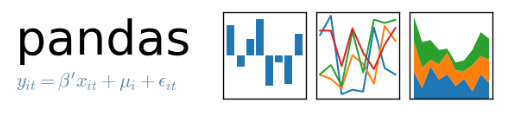[技术干货] 分类与预测——支持向量机

# 【Python算法】分类与预测——支持向量机

1.支持向量机定义
在机器学习领域，支持向量机 SVM（Support Vector Machine）是一个有监督的学习模型，通常用来进行模式识别、分类、以及回归分析。给出一个简单的线性分类问题，要用一条直线，将下图 13.13 中圆形的点和三角形的点分开，这样的直线有无数条，例如图中画出的两条线都能进行分类。这些将类别分离的曲线称为超平面。已有的训练数据中，每个元素距离分离超平面都有一个距离。在添加超平面的时候，尽可能的使最靠近分离超平面的那个元素与超平面的距离变大。这样，加入新的数据的时候，分的准的概率会最大化，这便是 SVM 的主要思想。如下图所示。最大化间隔的流程主要如下：首先进行归一化，归一化的目的是除掉取值尺度的影响；其次，对所有元素求到超平面的欧氏距离，给定一个超平面 P，计算所有样本与超平面 P 的欧式距离，这其中最小的距离记为DP，SVM 的作用就是找到DP最大的超平面。下图中的虚线画出了最大间隔，那么在这两条虚线上的样本点就称为支持向量。上面介绍的是线性可分的情形，而在实际中，编程者经常会遇到线性不可分的样例，如下图中的一维数轴上的叉形和菱形点，无法简单地找到一个超平面将其分类。此时常用的做法是样本特征映射到高维空间去。如果将原始的一维特征空间映射到二维特征空间，那么就可以找到分离超平面，小于0时，就可以判别类别为1。当大于0时，就可以判别类别为0，如下图所示。线性不可分映射到高维空间，可能会导致维度非常高，计算复杂。核函数的价值在于它虽然也是讲特征进行从低维到高维的转换，但核函数事先在低维上进行计算，而将实质上的分类效果表现在高维上，也避免了直接在高维空间中的复杂计算。

2.支持向量机优缺点
支持向量机算法的优点主要如下：
(1) 在高维空间有效；
(2) 在特征维数高于样本数的时候仍然有效；
(3) 增、删非支持向量样本对模型没有影响；
(4) 有些成功的应用中，SVM 方法对核的选取不敏感。当然，支持向量机算法也存在一些缺陷，例如对于每个高维空间在此空间的映射 F，如何确定 F 映射，现在还没有合适的方法，所以对于一般的问题，SVM 只是把高维空间的复杂性的困难转为了求核函数的困难。而且即使确定核函数以后，在求解问题分类时，也要求解函数的二次规划，这就需要大量的存储空间。这也是 SVM 的一个问题。

3.操作系统

操作机：Linux_Ubuntu
操作机默认用户：root

4.实验工具Python是一种计算机程序设计语言。是一种动态的、面向对象的脚本语言，最初被设计用于编写自动化脚本(shell)，随着版本的不断更新和语言新功能的添加，越来越多被用于独立的、大型项目的开发。Python已经成为最受欢迎的程序设计语言之一。自从2004年以后，python的使用率呈线性增长。2011年1月，它被TIOBE编程语言排行榜评为2010年度语言。
由于Python语言的简洁性、易读性以及可扩展性，在国外用Python做科学计算的研究机构日益增多，一些知名大学已经采用Python来教授程序设计课程。例如卡耐基梅隆大学的编程基础、麻省理工学院的计算机科学及编程导论就使用Python语言讲授。

众多开源的科学计算软件包都提供了Python的调用接口，例如著名的计算机视觉库OpenCV、三维可视化库VTK、医学图像处理库ITK。而Python专用的科学计算扩展库就更多了，例如如下3个十分经典的科学计算扩展库：NumPy、SciPy和matplotlib，它们分别为Python提供了快速数组处理、数值运算以及绘图功能。因此Python语言及其众多的扩展库所构成的开发环境十分适合工程技术、科研人员处理实验数据、制作图表，甚至开发科学计算应用程序。

5.NumpyNumPy系统是Python的一种开源的数值计算扩展。这种工具可用来存储和处理大型矩阵，比Python自身的嵌套列表（nested list structure)结构要高效的多（该结构也可以用来表示矩阵（matrix））。
NumPy（Numeric Python）提供了许多高级的数值编程工具，如：矩阵数据类型、矢量处理，以及精密的运算库。专为进行严格的数字处理而产生。多为很多大型金融公司使用，以及核心的科学计算组织如：Lawrence Livermore，NASA用其处理一些本来使用C++，Fortran或Matlab等所做的任务。

6.scikit-learnscikit-learn，Python 中的机器学习，简单高效的数据挖掘和数据分析工具，可供大家使用，可在各种环境中重复使用，建立在 NumPy，SciPy 和 matplotlib 上开放源码，可商业使用 - BSD license。

7.MatplotlibMatplotlib 是一个 Python 的 2D绘**，它以各种硬拷贝格式和跨平台的交互式环境生成出版质量级别的图形。通过 Matplotlib，开发者可以仅需要几行代码，便可以生成绘图，直方图，功率谱，条形图，错误图，散点图等。

8.pandasPython Data Analysis Library 或 pandas 是基于NumPy 的一种工具，该工具是为了解决数据分析任务而创建的。Pandas 纳入了大量库和一些标准的数据模型，提供了高效地操作大型数据集所需的工具。pandas提供了大量能使我们快速便捷地处理数据的函数和方法。你很快就会发现，它是使Python成为强大而高效的数据分析环境的重要因素之一。

9.导入所需要的库:numpy,matplotlib.pyplot,pandas

```# Importing the libraries
import numpy as np
import matplotlib.pyplot as plt
import pandas as pd10.加载数据集```

```# Importing the dataset
dataset = pd.read_csv('/mnt/dataset_29/Social_Network_Ads_82e4aa0627105a2d22b70d7ad0bfeda0.csv')   注意：数据集以实际位置为准
X = dataset.iloc[:, [2, 3]].values
y = dataset.iloc[:, 4].values```11.将数据集分割为训练集和测试集

```# Splitting the dataset into the Training set and Test set
from sklearn.model_selection import train_test_split
X_train, X_test, y_train, y_test = train_test_split(X, y, test_size = 0.25, random_state = 0)```

12.特征缩放

```# Feature Scaling
from sklearn.preprocessing import StandardScaler
sc = StandardScaler()
X_train = sc.fit_transform(X_train)
X_test = sc.transform(X_test)```

``

13.使用Kernel SVM对数据进行处理

```# Fitting Kernel SVM to the Training set
from sklearn.svm import SVC
classifier = SVC(kernel = 'rbf', random_state = 0)
classifier.fit(X_train, y_train)```14.对测试集进行分类

```# Predicting the Test set results
y_pred = classifier.predict(X_test)```

15.制作混淆矩阵来评估模型性能

```# Making the Confusion Matrix
from sklearn.metrics import confusion_matrix
cm = confusion_matrix(y_test, y_pred)```

16.绘制训练数据分类结果

```# Visualising the Training set results
from matplotlib.colors import ListedColormap
X_set, y_set = X_train, y_train
X1, X2 = np.meshgrid(np.arange(start = X_set[:, 0].min() - 1, stop = X_set[:, 0].max() + 1, step = 0.01),
np.arange(start = X_set[:, 1].min() - 1, stop = X_set[:, 1].max() + 1, step = 0.01))
plt.contourf(X1, X2, classifier.predict(np.array([X1.ravel(), X2.ravel()]).T).reshape(X1.shape),
alpha = 0.75, cmap = ListedColormap(('red', 'green')))
plt.xlim(X1.min(), X1.max())
plt.ylim(X2.min(), X2.max())
for i, j in enumerate(np.unique(y_set)):
plt.scatter(X_set[y_set == j, 0], X_set[y_set == j, 1],
c = ListedColormap(('orange', 'blue'))(i), label = j)
plt.title('Classifier (Training set)')
plt.xlabel('Age')
plt.ylabel('Estimated Salary')
plt.legend()
plt.show()```

``

17.绘制测试数据分类结果

```# Visualising the Test set results
from matplotlib.colors import ListedColormap
X_set, y_set = X_test, y_test
X1, X2 = np.meshgrid(np.arange(start = X_set[:, 0].min() - 1, stop = X_set[:, 0].max() + 1, step = 0.01),
np.arange(start = X_set[:, 1].min() - 1, stop = X_set[:, 1].max() + 1, step = 0.01))
plt.contourf(X1, X2, classifier.predict(np.array([X1.ravel(), X2.ravel()]).T).reshape(X1.shape),
alpha = 0.75, cmap = ListedColormap(('red', 'green')))
plt.xlim(X1.min(), X1.max())
plt.ylim(X2.min(), X2.max())
for i, j in enumerate(np.unique(y_set)):
plt.scatter(X_set[y_set == j, 0], X_set[y_set == j, 1],
c = ListedColormap(('orange', 'blue'))(i), label = j)
plt.title('Classifier (Test set)')
plt.xlabel('Age')
plt.ylabel('Estimated Salary')
plt.legend()
plt.show()```

``